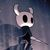# New to Qlik Sense

If you’re new to Qlik Sense, start with this Discussion Board and get up-to-speed quickly.

Announcements
QlikWorld 2023, a live, in-person thrill ride. Save \$300 before February 6: REGISTER NOW!
cancel
Showing results for
Did you mean:Contributor II

## Compare same periods different years

I want to compare different years but same periods, so it would be for 2020 until today and same period for 2019

For 2020 I have this formula:

Sum({<[Fecha - hora.autoCalendar.Year]= {\$(=Max(Year([Fecha - hora.autoCalendar.Year])))}>}[Total])

For 2019 I have this formula:

Sum({<[Fecha - hora.autoCalendar.Year]= {\$(=Max(Year([Fecha - hora.autoCalendar.Year]-1)))}>}[Total])

How do I compare two years but for the same period of time as the Max(Year([today])

Labels (1)
• ### Compare same periods different years

1 Solution

Accepted SolutionsContributor III

Try using this formulas for CY YTD and PY YTD: based on your date_column format change the date format to make it work.

CY YTD:

SUM({\$<DATE_COLUMN={">=\$(=DATE(YearStart(TODAY()),'YYYY-MM-DD'))<=\$(=DATE(TODAY(),'YYYY-MM-DD'))"}>}TOTAL)

PY YTD:

hope this helps

2 RepliesPartner - Creator II

Hello,

You're interested in a year-to-date analysis.Contributor III

Try using this formulas for CY YTD and PY YTD: based on your date_column format change the date format to make it work.

CY YTD:

SUM({\$<DATE_COLUMN={">=\$(=DATE(YearStart(TODAY()),'YYYY-MM-DD'))<=\$(=DATE(TODAY(),'YYYY-MM-DD'))"}>}TOTAL)

PY YTD: